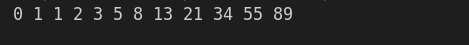The Fibonacci sequence is a series where the next term is the sum of the previous two terms. The first two terms of the Fibonacci sequence are 0 and 1.

The series is as follows: 0, 1, 1, 2, 3, 5, 8, 13, 21, 34, …

Write a C++ program (using loop) to print the 10 numbers of the Fibonacci series as shown above.

#include <iostream>
using namespace std;

// 0 1 1 2 3 5 8 13 21 34 55 89

int main()
{
int number1 = 0;
int number2 = 1;
cout<<number1<<" "<<number2<<" ";
for (int i = 1; i <= 5; i++)
{
number1 += number2;
number2 += number1;
cout<<number1<<" "<<number2<<" ";
}

return 0;
}cpp programming exercise with solution